# Reasoning Under Uncertainty Belief Networks Computer Science cpsc

• Slides: 26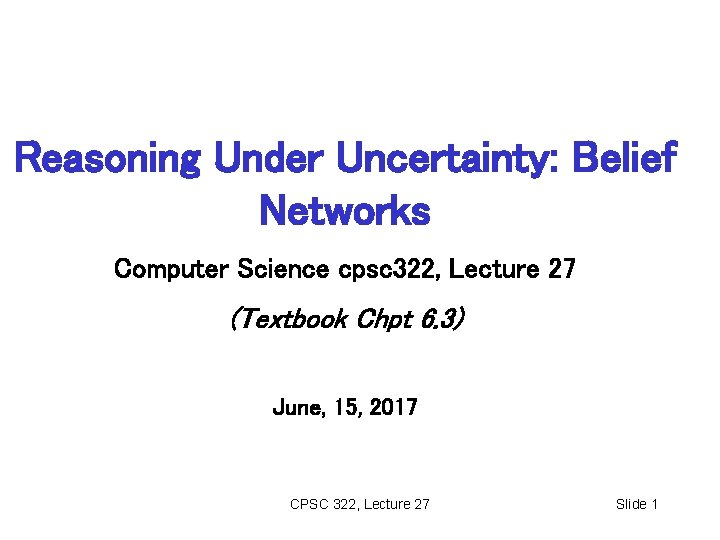Reasoning Under Uncertainty: Belief Networks Computer Science cpsc 322, Lecture 27 (Textbook Chpt 6. 3) June, 15, 2017 CPSC 322, Lecture 27 Slide 1Big Picture: R&R systems Environment Problem Static Deterministic Arc Consistency Search Constraint Vars + Satisfaction Constraints Stochastic SLS Belief Nets Query Logics Search Sequential Planning Representation Reasoning Technique STRIPS Search Var. Elimination Decision Nets Var. Elimination Markov Processes Value Iteration CPSC 322, Lecture 27 Slide 2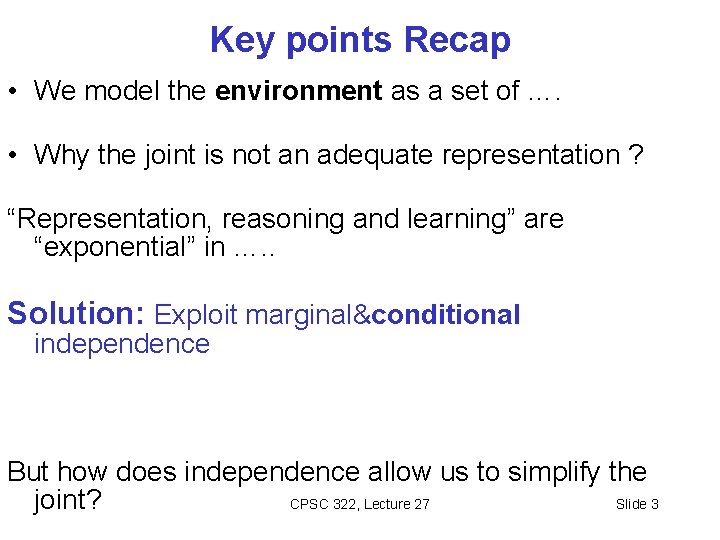Key points Recap • We model the environment as a set of …. • Why the joint is not an adequate representation ? “Representation, reasoning and learning” are “exponential” in …. . Solution: Exploit marginal&conditional independence But how does independence allow us to simplify the CPSC 322, Lecture 27 Slide 3 joint?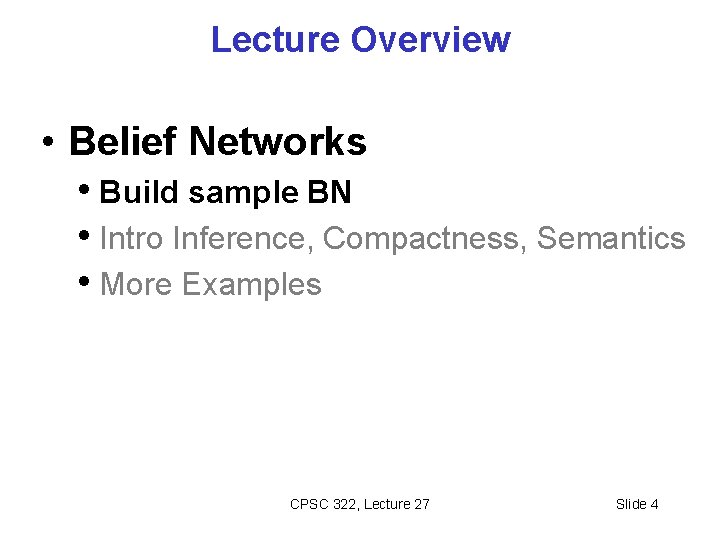Lecture Overview • Belief Networks • Build sample BN • Intro Inference, Compactness, Semantics • More Examples CPSC 322, Lecture 27 Slide 4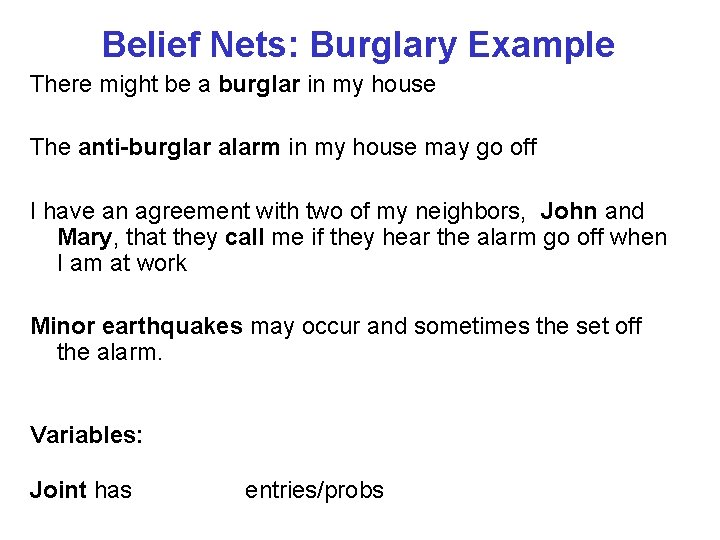Belief Nets: Burglary Example There might be a burglar in my house The anti-burglar alarm in my house may go off I have an agreement with two of my neighbors, John and Mary, that they call me if they hear the alarm go off when I am at work Minor earthquakes may occur and sometimes the set off the alarm. Variables: Joint has entries/probs CPSC 322, Lecture 27 Slide 5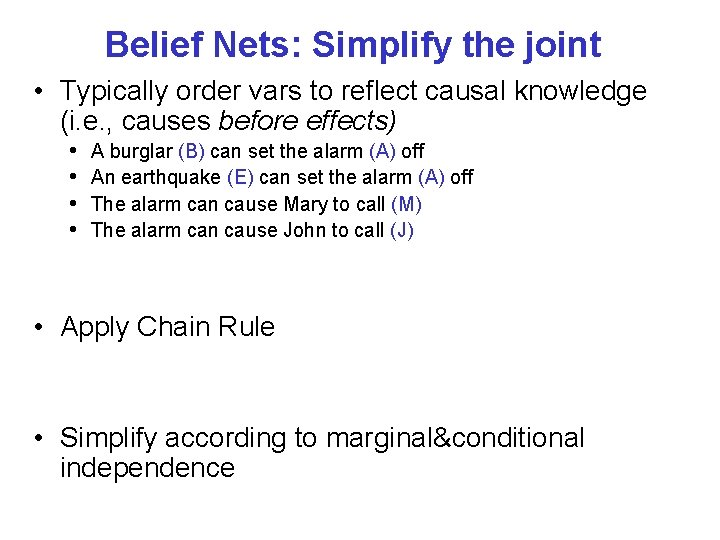Belief Nets: Simplify the joint • Typically order vars to reflect causal knowledge (i. e. , causes before effects) • • A burglar (B) can set the alarm (A) off An earthquake (E) can set the alarm (A) off The alarm can cause Mary to call (M) The alarm can cause John to call (J) • Apply Chain Rule • Simplify according to marginal&conditional independence CPSC 322, Lecture 27 Slide 6Belief Nets: Structure + Probs • Express remaining dependencies as a network • Each var is a node • For each var, the conditioning vars are its parents • Associate to each node corresponding conditional probabilities CPSC 322, Lecture 27 • Directed Acyclic Graph (DAG) Slide 7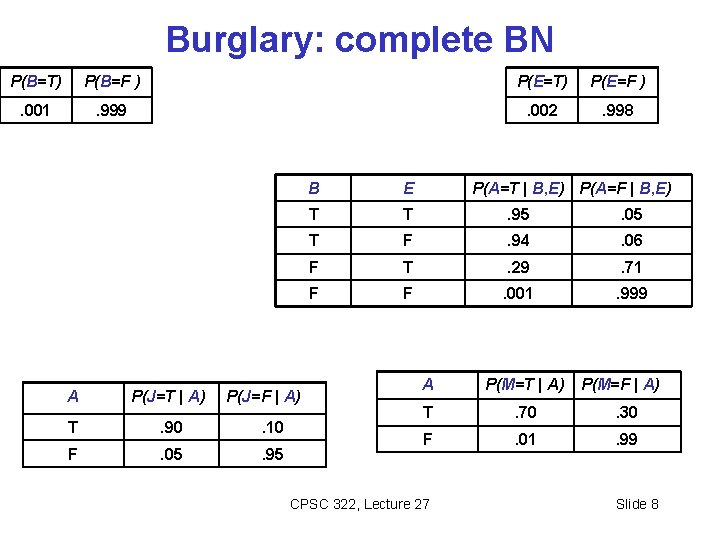Burglary: complete BN P(B=T) P(B=F ) P(E=T) P(E=F ) . 001 . 999 . 002 . 998 A P(J=T | A) P(J=F | A) T . 90 . 10 F . 05 . 95 B E P(A=T | B, E) P(A=F | B, E) T T . 95 . 05 T F . 94 . 06 F T . 29 . 71 F F . 001 . 999 A P(M=T | A) T . 70 . 30 F . 01 . 99 CPSC 322, Lecture 27 P(M=F | A) Slide 8Lecture Overview • Belief Networks • Build sample BN • Intro Inference, Compactness, Semantics • More Examples CPSC 322, Lecture 27 Slide 9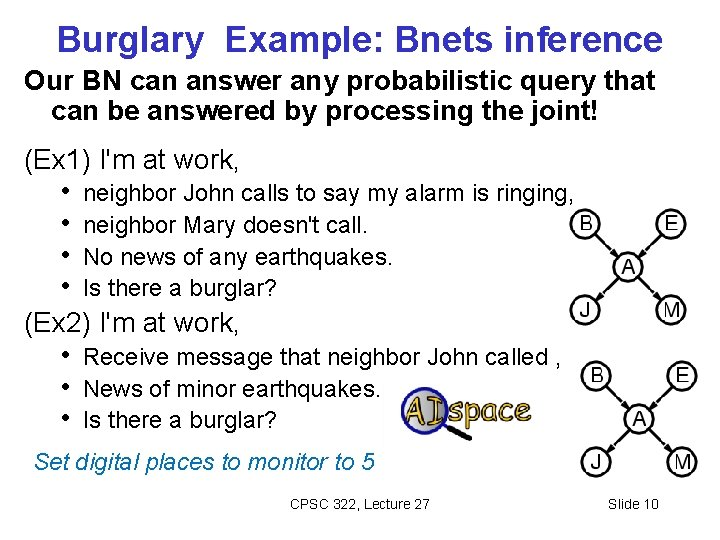Burglary Example: Bnets inference Our BN can answer any probabilistic query that can be answered by processing the joint! (Ex 1) I'm at work, • neighbor John calls to say my alarm is ringing, • neighbor Mary doesn't call. • No news of any earthquakes. • Is there a burglar? (Ex 2) I'm at work, • Receive message that neighbor John called , • News of minor earthquakes. • Is there a burglar? Set digital places to monitor to 5 CPSC 322, Lecture 27 Slide 10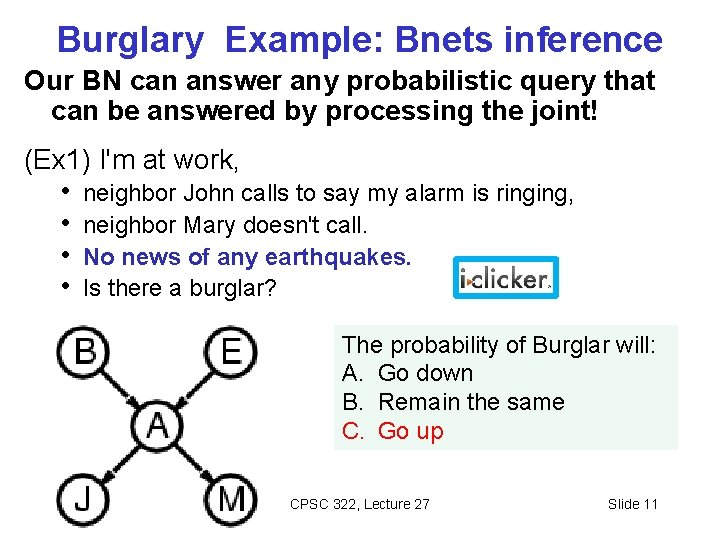Burglary Example: Bnets inference Our BN can answer any probabilistic query that can be answered by processing the joint! (Ex 1) I'm at work, • neighbor John calls to say my alarm is ringing, • neighbor Mary doesn't call. • No news of any earthquakes. • Is there a burglar? The probability of Burglar will: A. Go down B. Remain the same C. Go up CPSC 322, Lecture 27 Slide 11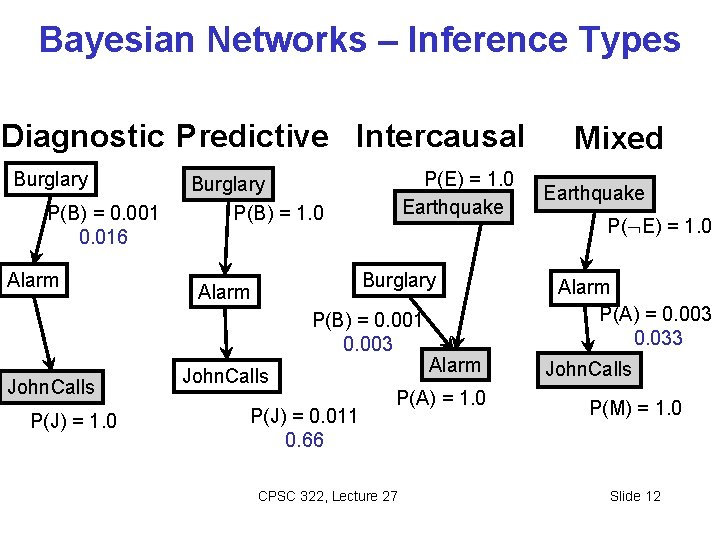Bayesian Networks – Inference Types Diagnostic Predictive Intercausal Burglary P(B) = 0. 001 0. 016 Alarm P(E) = 1. 0 Earthquake Burglary P(B) = 1. 0 Burglary Alarm P(B) = 0. 001 0. 003 John. Calls P(J) = 1. 0 John. Calls P(J) = 0. 011 0. 66 Alarm P(A) = 1. 0 CPSC 322, Lecture 27 Mixed Earthquake P( E) = 1. 0 Alarm P(A) = 0. 003 0. 033 John. Calls P(M) = 1. 0 Slide 12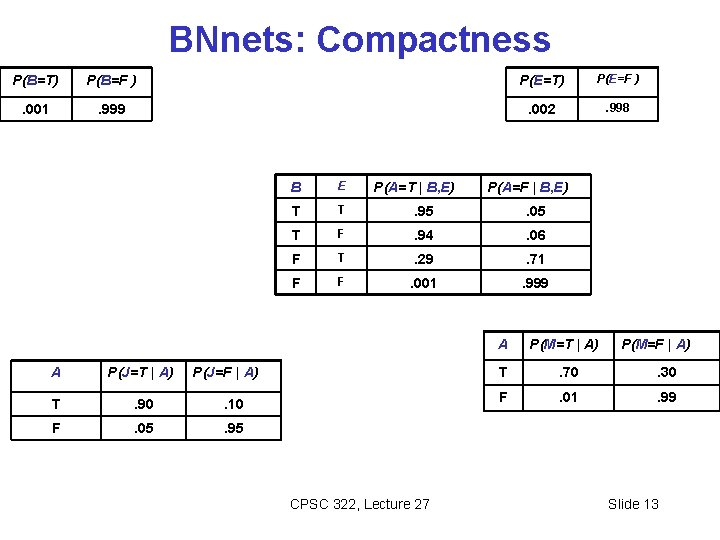BNnets: Compactness P(B=T) P(B=F ) P(E=T) P(E=F ) . 001 . 999 . 002 . 998 A P(J=T | A) T . 90 . 10 F . 05 . 95 B E T T . 95 . 05 T F . 94 . 06 F T . 29 . 71 F F . 001 . 999 P(A=T | B, E) P(J=F | A) CPSC 322, Lecture 27 P(A=F | B, E) A P(M=T | A) P(M=F | A) T . 70 . 30 F . 01 . 99 Slide 13BNets: Compactness In General: A CPT for boolean Xi with k boolean parents has the combinations of parent values Each row requires one number pi for Xi = true (the number for Xi = false is just 1 -pi ) rows for If each variable has no more than k parents, the complete network requires O( ) numbers For k<< n, this is a substantial improvement, • the numbers required grow linearly with n, vs. O(2 n) for the full joint distribution CPSC 322, Lecture 27 Slide 14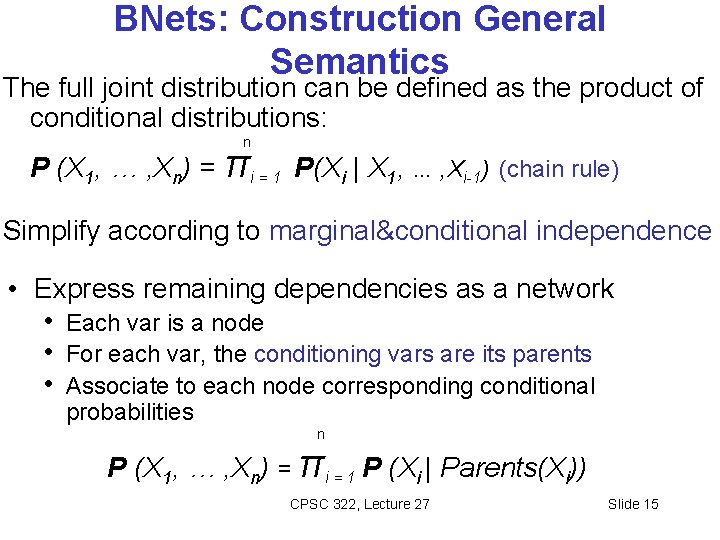BNets: Construction General Semantics The full joint distribution can be defined as the product of conditional distributions: n P (X 1, … , Xn) = πi = 1 P(Xi | X 1, … , Xi-1) (chain rule) Simplify according to marginal&conditional independence • Express remaining dependencies as a network • Each var is a node • For each var, the conditioning vars are its parents • Associate to each node corresponding conditional probabilities n P (X 1, … , Xn) = πi = 1 P (Xi | Parents(Xi)) CPSC 322, Lecture 27 Slide 15BNets: Construction General Semantics (cont’) n P (X 1, … , Xn) = πi = 1 P (Xi | Parents(Xi)) • Every node is independent from its non-descendants given it parents CPSC 322, Lecture 27 Slide 16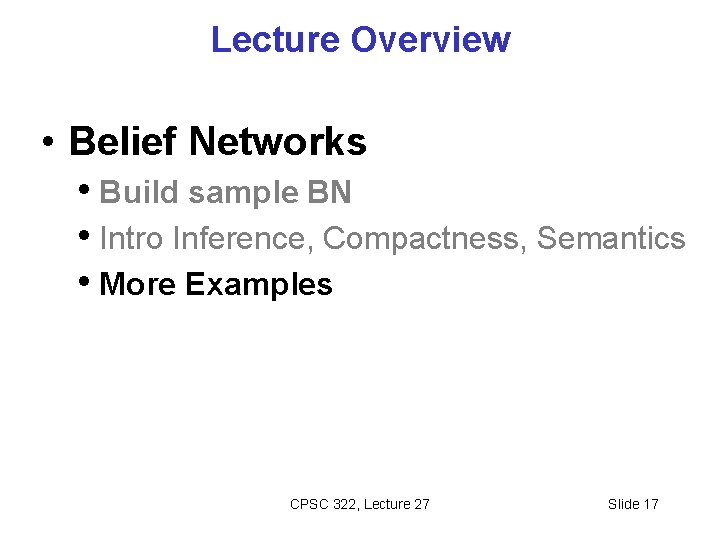Lecture Overview • Belief Networks • Build sample BN • Intro Inference, Compactness, Semantics • More Examples CPSC 322, Lecture 27 Slide 17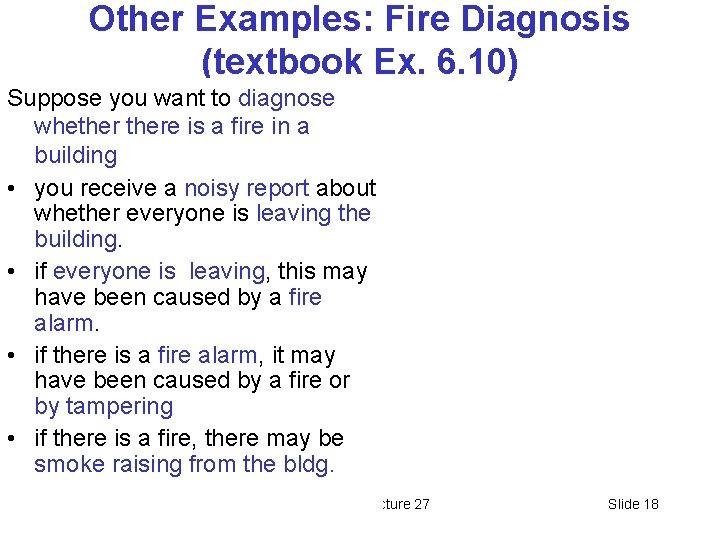Other Examples: Fire Diagnosis (textbook Ex. 6. 10) Suppose you want to diagnose whethere is a fire in a building • you receive a noisy report about whether everyone is leaving the building. • if everyone is leaving, this may have been caused by a fire alarm. • if there is a fire alarm, it may have been caused by a fire or by tampering • if there is a fire, there may be smoke raising from the bldg. CPSC 322, Lecture 27 Slide 18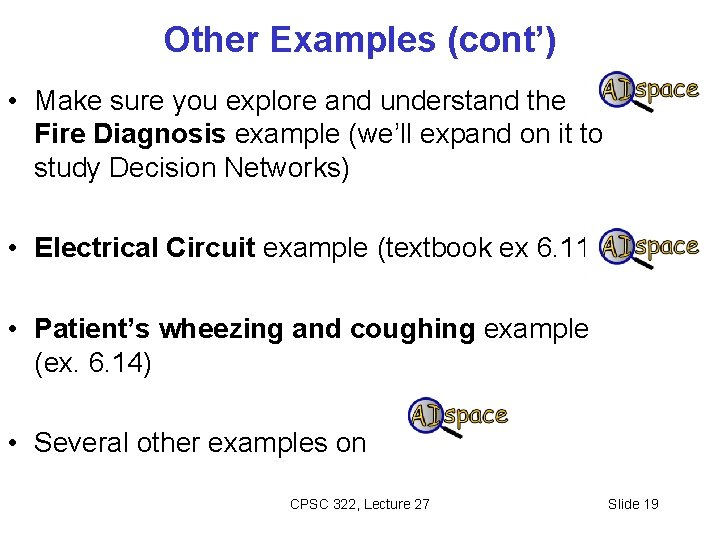Other Examples (cont’) • Make sure you explore and understand the Fire Diagnosis example (we’ll expand on it to study Decision Networks) • Electrical Circuit example (textbook ex 6. 11) • Patient’s wheezing and coughing example (ex. 6. 14) • Several other examples on CPSC 322, Lecture 27 Slide 19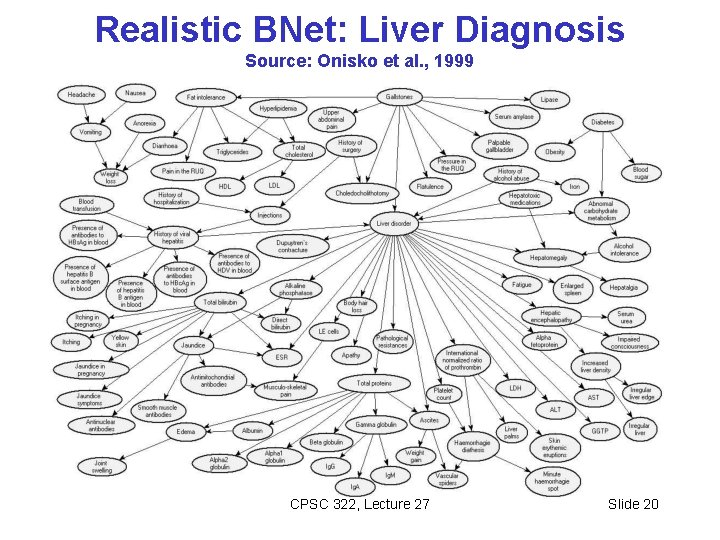Realistic BNet: Liver Diagnosis Source: Onisko et al. , 1999 CPSC 322, Lecture 27 Slide 20Realistic BNet: Liver Diagnosis Source: Onisko et al. , 1999 CPSC 322, Lecture 27 Slide 21Realistic BNet: Liver Diagnosis Source: Onisko et al. , 1999 Assuming there are ~60 nodes in this Bnet with max number of parents =4; and assuming all nodes are binary, how many numbers are required for the JPD vs BNet JPD BNet A ~1018 ~103 B ~1030 ~1018 C ~1013 ~1014 D ~103 CPSC 322, Lecture 27 Slide 22Answering Query under Uncertainty Probability Theory Static Belief Network & Variable Elimination Dynamic Bayesian Network Hidden Markov Models Monitoring (e. g credit cards) Diagnostic Systems (e. g. , medicine) Student Tracing in tutoring Systems Natural Language Processing Email spam filters CPSC 322, Lecture 27 Slide 23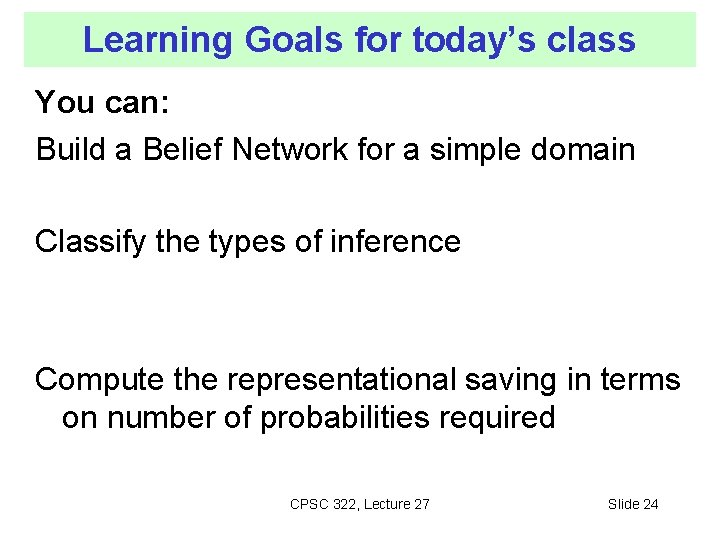Learning Goals for today’s class You can: Build a Belief Network for a simple domain Classify the types of inference Compute the representational saving in terms on number of probabilities required CPSC 322, Lecture 27 Slide 24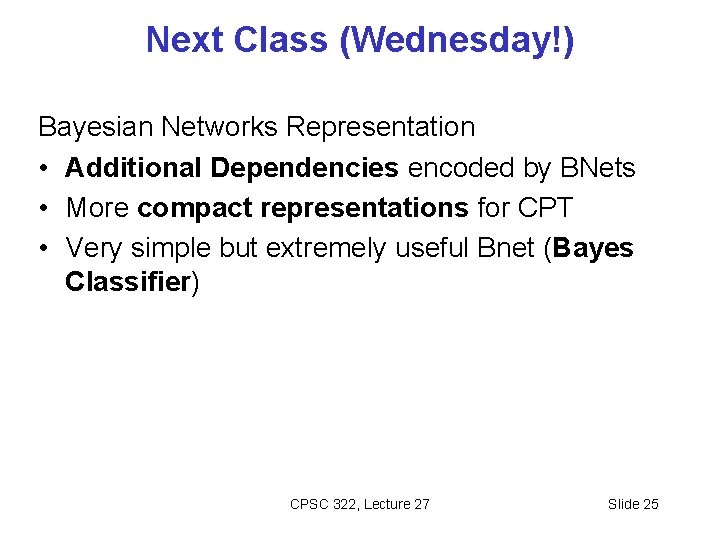Next Class (Wednesday!) Bayesian Networks Representation • Additional Dependencies encoded by BNets • More compact representations for CPT • Very simple but extremely useful Bnet (Bayes Classifier) CPSC 322, Lecture 27 Slide 25Belief network summary • A belief network is a directed acyclic graph (DAG) that effectively expresses independence assertions among random variables. • The parents of a node X are those variables on which X directly depends. • Consideration of causal dependencies among variables typically help in constructing a Bnet CPSC 322, Lecture 27 Slide 26